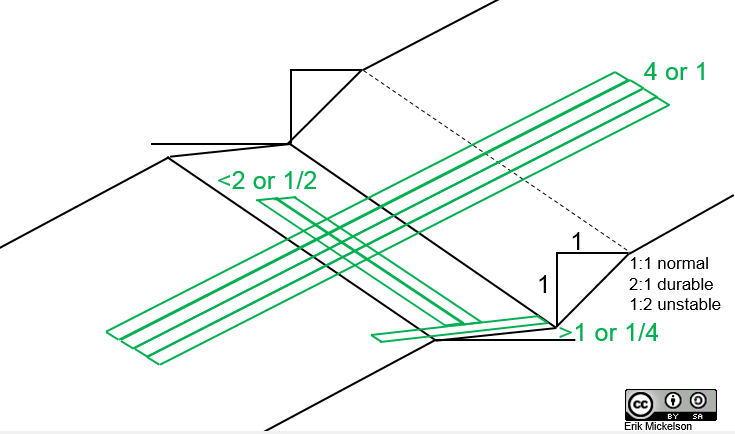Trailism

# Trail Math

A short and incomplete list of equations and calculators useful for trails.

#### Layout Equations$\%Slope=\frac{Rise}{Run}\times100$$Slope\textdegree=(\frac{Rise}{Run})\tan^{-1}$

or$Slope\textdegree=(\frac{\theta\%}{100})\tan^{-1}$$Rise\:or\:Fall=b=(Running\:Grade)\sin\theta\textdegree$

note: Forest Service Trail Accessibility Guidelines (FSTAG) 7.4.3.2 “The cross slope [out slope] shall not exceed 1:20 (5 percent). Where the surface is paved or is elevated above the natural ground, the cross slope shall not be steeper than 1:48 (2 percent).”

### Half Rulein actuality the half rule is really equal to and less than…but it’s really not a “rule” as much as a suggestion$Half\;Rule=Slope\;Grade\leqslant\frac{Fall\;Line}{2}$$OutSlope\;Half\;Rule=OutSlope\;Grade\geqslant\frac{Fall\;Line}{4}$

or$OutSlope\;Half\;Rule=OutSlope\;Grade\geqslant\frac{Running\;Grade}{2}$

### Trail Length

A rough estimate of the trail length for a segment based on elevation gain or loss (typically the average grade should be less than 10%):$Trail\;Distance=\frac{Elevation\;Change}{Desired\;\%Grade\;as\;decimal}$

### Turns$180\textdegree\;Turn\;Length= Circumference\;/2=(2\pi\times r)/2$$180\textdegree\;Turn\;Grade= (\%Slope\;Turn\;Descends/100)tan^{-1})/(\pi/2)$

180° Turn Grade? Rise/Run @ Diameter vs. Rise/Run @ Circumference:

#### More on the complicated machinations of turning on a bike:

I hope to publish some nomograms in the future for some of the above math applications on this and other pages.

Scroll to Top